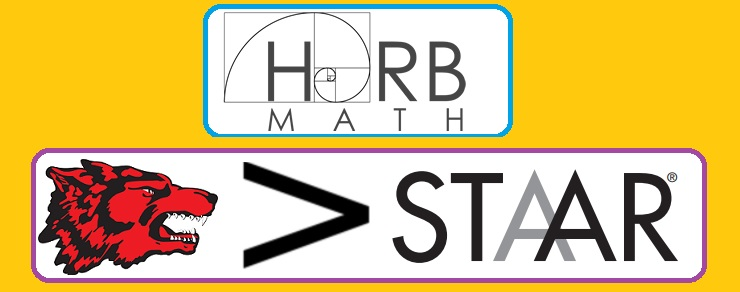# ⛧ Q 29 GR : System of equations given one line and one equation or points⛧

10 Questions
50 Minutes
⛧ Q 29- STAAR 2017⛧
GR : System of equations given one line and one equation or points

School ID